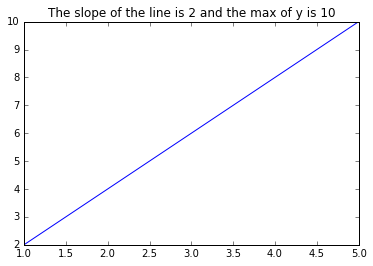#### matplotlib.pyplot画图的标题中插入变量

统计/机器学习 数据可视化 Python    浏览次数：13390        分享

2

import matplotlib.pyplot as plt

plt.plot(x, y)

plt.tittle("Results of Iteration k")小菜   2017-04-10 20:17

##### 1个回答
2

import numpy as np

import matplotlib.pyplot as plt

k = 2

x = np.array([1, 2, 3, 4, 5])

y = k * x

plt.figure()

plt.plot(x, y)

plt.title("The slope of the line is %s and the max of y is %s"%(k, max(y)))SofaSofa数据科学社区DS面试题库 DS面经派大星   2017-04-12 08:40相关主题

python画散点图   1回答